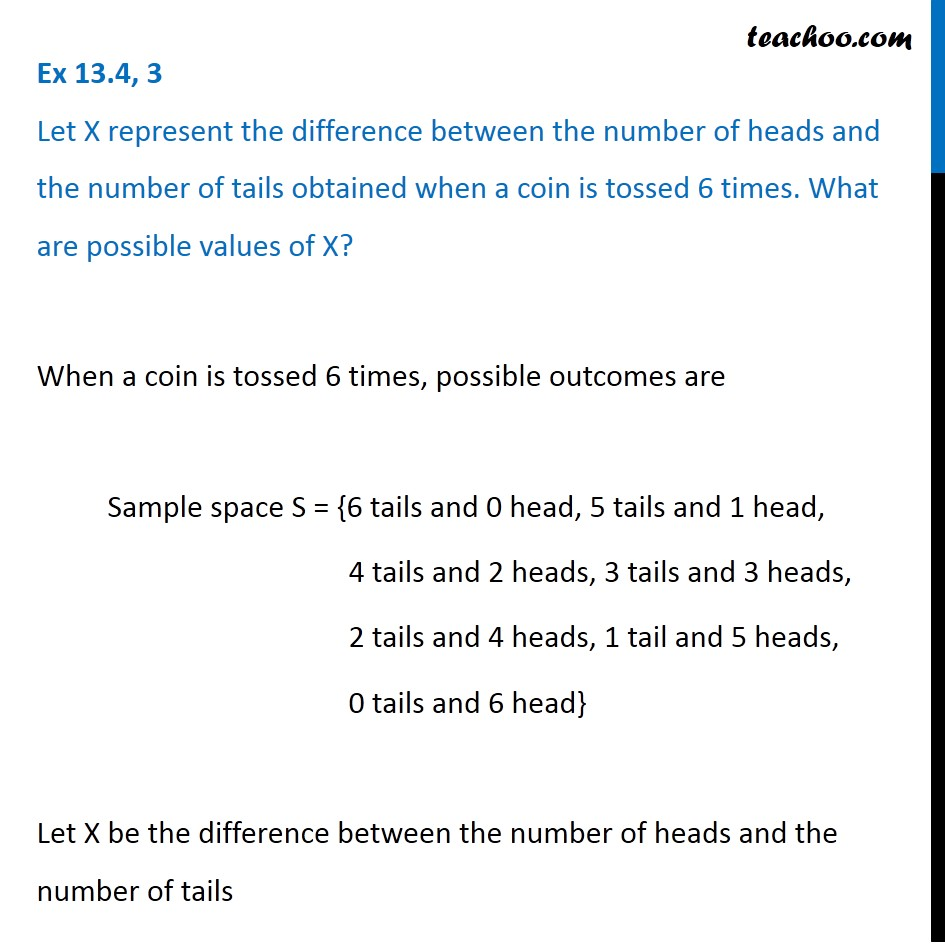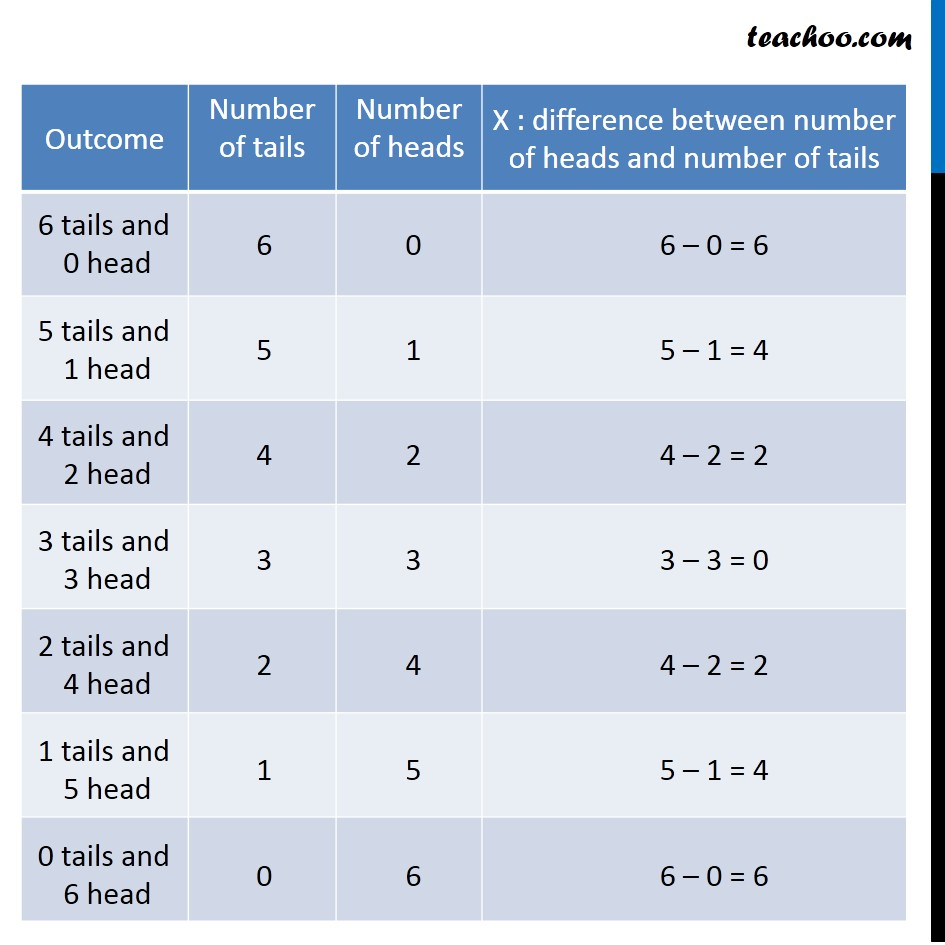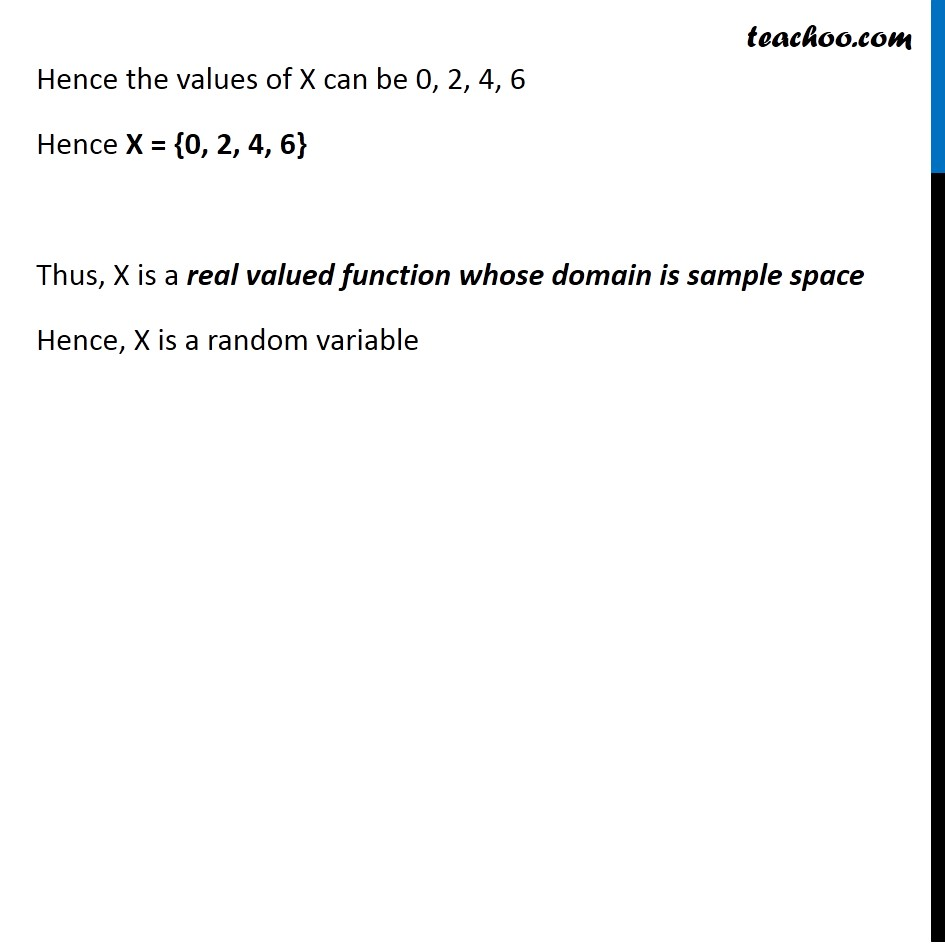1. Chapter 13 Class 12 Probability (Term 2)
2. Serial order wise
3. Ex 13.4

Transcript

Ex 13.4, 3 Let X represent the difference between the number of heads and the number of tails obtained when a coin is tossed 6 times. What are possible values of X?When a coin is tossed 6 times, possible outcomes are Sample space S = {6 tails and 0 head, 5 tails and 1 head, 4 tails and 2 heads, 3 tails and 3 heads, 2 tails and 4 heads, 1 tail and 5 heads, 0 tails and 6 head} Let X be the difference between the number of heads and the number of tails 6 tails and 0 head Hence the values of X can be 0, 2, 4, 6 Hence X = {0, 2, 4, 6} Thus, X is a real valued function whose domain is sample space Hence, X is a random variable Ex 13.4, 4 Find the probability distribution of (i) number of heads in two tosses of a coin. Let X: Number of heads We toss coin twice So, we can get 0 heads, 1 heads or 2 heads. So, value of X can be 0, 1, 2 So the Probability distribution

Ex 13.4